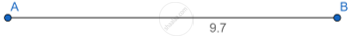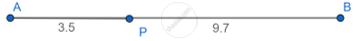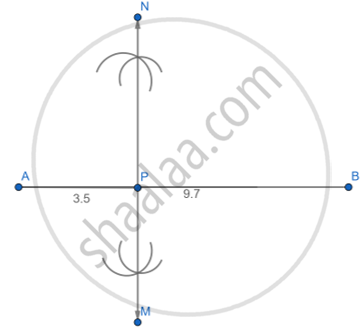# Draw Seg Ab of Length 9.7 Cm. Take a Point P on It Such that A-p-b, Ap = 3.5 Cm. Construct a Line Mn Sag Ab Through Point P. - Geometry

Diagram

Draw seg AB of length 9.7 cm. Take a point P on it such that A-P-B, AP = 3.5 cm. Construct a line MNsag AB through point P.

#### Solution

Steps of construction:

i: Draw line segment AB of 9.7 cm.ii: Take point P on such that AP = 3.5cmiii: Construct a line segment MN  AB through point P.Concept: Division of a Line Segment
Is there an error in this question or solution?